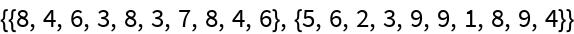# BisectList

Contributed by: George Beck

Make a pair of lists consisting of alternate terms from the original list

 ResourceFunction["BisectList"][{a1,a2,a3,a4,a5,a6,…}] makes the list {{a1,a3,a5…},{a2,a4,a4,…}}.

## Details and Options

ResourceFunction["BisectList"] is a partial inverse function of Riffle.
The two sublists have equal lengths.
If the input list has an odd number of terms, the last term is ignored.
The argument can be an expression other than a list.

## Examples

### Basic Examples

The lists being riffled have the same length:

 In:=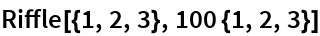Out=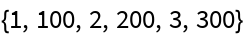BisectList gives back the sublists:

 In:=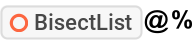Out=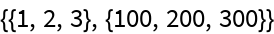Here the riffled lists have different lengths:

 In:=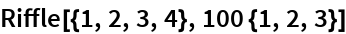Out=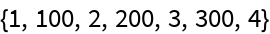BisectList ignores the last term:

 In:=Out=Here the head of the expression is not a list:

 In:=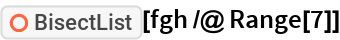Out=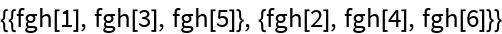### Scope

Add consecutive pairs of a list:

 In:=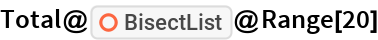Out=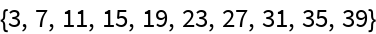Apply BisectList twice:

 In:=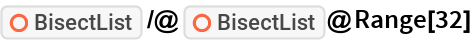Out=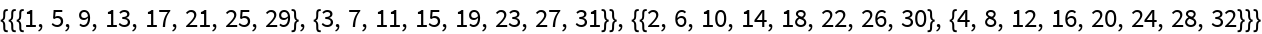Pull out the interleaved subsequences, making them both positive:

 In:=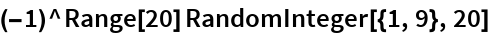Out=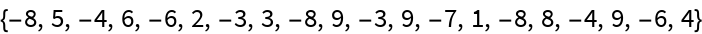In:=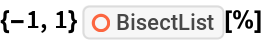Out=